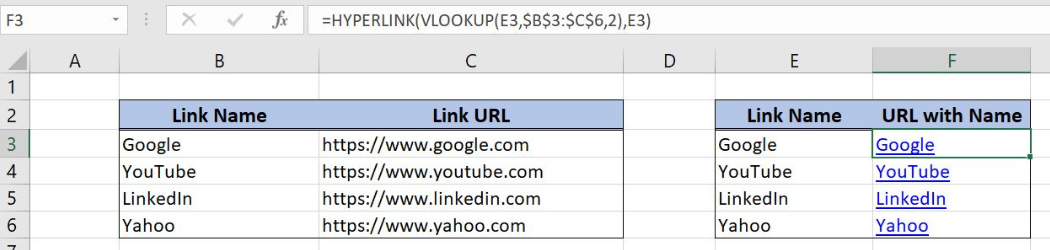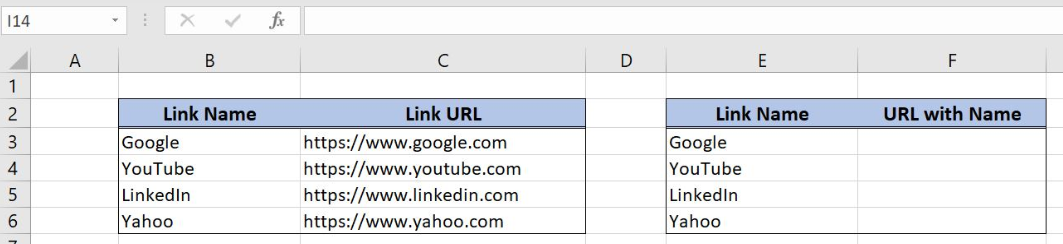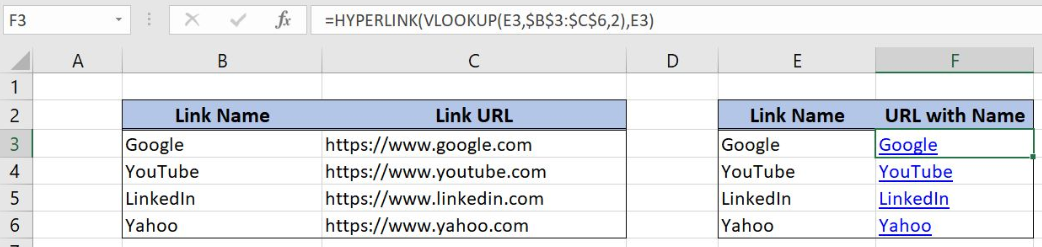Get instant live expert help with Excel or Google Sheets“My Excelchat expert helped me in less than 20 minutes, saving me what would have been 5 hours of work!”

#### Post your problem and you'll get expert help in seconds

Your message must be at least 40 characters
Our professional experts are available now. Your privacy is guaranteed.

Excel allows us to build a hyperlink with a name, using the HYPERLINK and VLOOKUP functions. This step by step tutorial will assist all levels of Excel users in creating a hyperlink from name and URL.Figure 1. The result of the HYPERLINK and VLOOKUP functions

## Syntax of the VLOOKUP formula

The generic formula of VLOOKUP looks like:

`=VLOOKUP(lookup_value, table_array, col_index_num, range_lookup)`

The parameters of the VLOOKUP function are:

• lookup_value – a value that we want to find in the VLOOKUP table
• table_array – a range in which we want to lookup
• col_index_num – a column number from which we would like to pull a value
• range_lookup – default value 0. This means that we want to find an exact match for a lookup value.

## Syntax of the HYPERLINK formula

The generic formula of HYPERLINK looks like:

`=HYPERLINK(link_location, friendly_name)`

The parameters of the HYPERLINK function are:

• table_array – a name for the hyperlink.

## Setting up Our Data for the Self-contained VLOOKUP Function

Let’s look at the structure of the data we will use. In the range B2:C6 we have the table from which we want to pull data. Column B has “Link Name” and column C has “Link URL”. In column F, we want to create a hyperlink with name, based on a name from the column E. Therefore, we first need to look up for the URL in the lookup table B2:C6.Figure 2. Data structure for the self-contained VLOOKUP

## Using the HYPERLINK and VLOOKUP Functions

In our example, we want to get get the Link URL from column C based on Link Name in E3 (Google). Based on that two values, we want to create a hyperlink with a name. The result is in the cell F3.

The formula looks like:

`=HYPERLINK(VLOOKUP(E3, \$B\$3:\$C\$6, 2), E3)`

The lookup_value is the cell E3. The parameter table_array is the range \$B\$3:\$C\$6. The range must be fixed, as it is not changing when the formula is copied. Col_index_num has value 2, as we want to pull value from the second column of the range. Finally, range_lookup has value 0 by default, because we want to find an exact match of “Lookup column” values.

The result of the VLOOKUP function is the link_location parameter for the HYPERLINK function. The friendly_name parameter is the cell E3.

To apply the HYPERLINK and VLOOKUP functions, we need to follow these steps:

• Select cell F3 and click on it
• Insert the formula: `=HYPERLINK(VLOOKUP(E3, \$B\$3:\$C\$6, 2), E3)`
• Press enter
• Drag the formula down to the other cells in the column by clicking and dragging the little “+” icon at the bottom-right of the cell.Figure 3. Application of the self-contained VLOOKUP formula

As a result, we will get the hyperlink for https://www.google.com with the name “Google” in the cell F3. We can see in the VLOOKUP table that this is the Link URL for the name Google from E3.

Most of the time, the problem you will need to solve will be more complex than a simple application of a formula or function. If you want to save hours of research and frustration, try our live Excelchat service! Our Excel Experts are available 24/7 to answer any Excel question you may have. We guarantee a connection within 30 seconds and a customized solution within 20 minutes.

### Did this post not answer your question? Get a solution from connecting with the expert.Another blog reader asked this question today on Excelchat:## Subscribe to Excelchat.coAnother blog reader asked this question today on Excelchat: